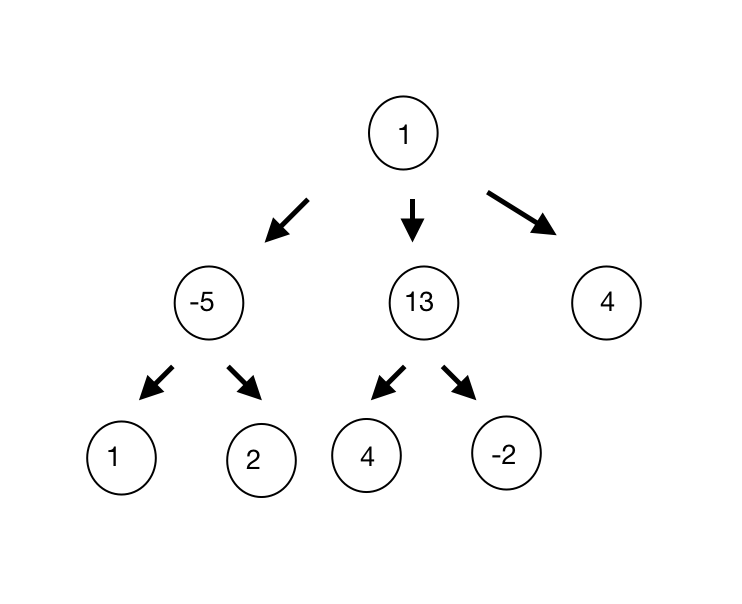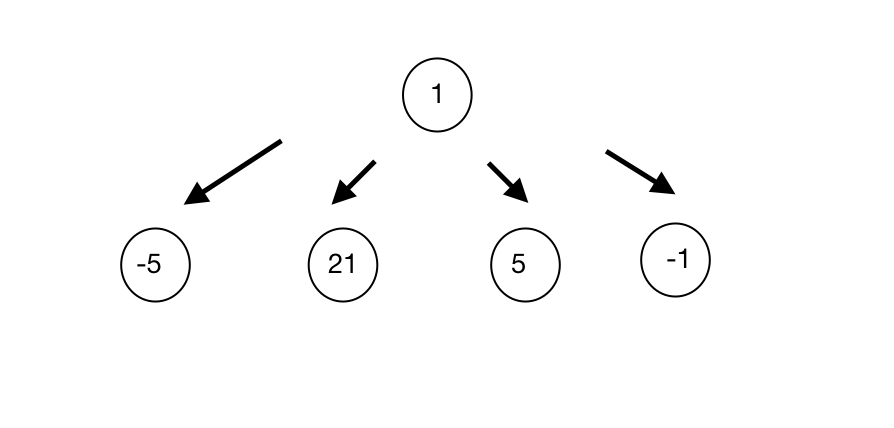## Interviews

#### [Amazon] Subtree: Maximum Average Node#### Subtree with Maximum Average

Given a M-ary tree, find the subtree with maximum average. Return the root of the subtree.
A subtree of a tree is any node of that tree plus all its descendants. The average value of a subtree is the sum of its values, divided by the number of nodes.

Example 1:
Input:Output: 13
Explanation: For the node with value = 13 we have an average of (13 + 4 + -2) / 3 = 5 which is the maximum.

Example 2:

Input:Output: 21
Explanation:
For the node with value = 1 we have an average of (- 5 + 21 + 5 - 1) / 5 = 4.
For the node with value = -5 we have an average of (-5 / 1) = -5.
For the node with value = 21 we have an average of (21 / 1) = 21.
For the node with value = 5 we have an average of (5 / 1) = 5.
For the node with value = -1 we have an average of (-1 / 1) = -1.
So the subtree of 21 is the maximum.

##### Solve the problem:

# Definition for a N-ary node. # class TreeNode: # def __init__(self, x): # self.val = x # self.children = [] # # Input type: TreeNode # Output type: TreeNode class TreeNode: def __init__(self, x): self.val = x self.children = [] def subtreeMaxAvg(root: TreeNode) -> TreeNode: